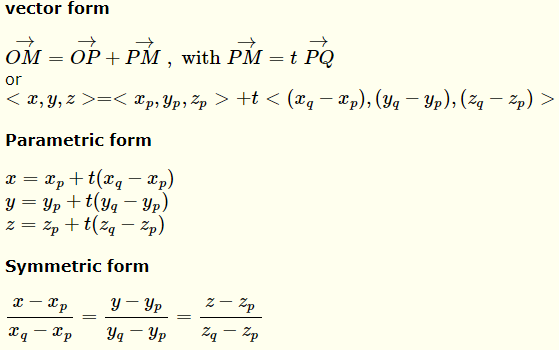# Equation of a Line Through two Points in 3 D


 A calculator and solver to find the equation of a line in vector, parametric and symmetric forms is presented. An unlimited number of examples may be generated interactively along with their solutions. Below is shown a line through two points $$P(x_p,y_p,z_p)$$ and $$Q(x_q,y_q,z_q)$$.Three forms of the equation of the line:Step by step solution STEP 1: Find the components of vetor $$\vec {PQ}$$ . STEP 2: Write Equation in Vector form. STEP 3: Write Equation in Parametric form. STEP 4: Write Equation in Symmetric form.
More Step by Step Math Worksheets Solvers New !